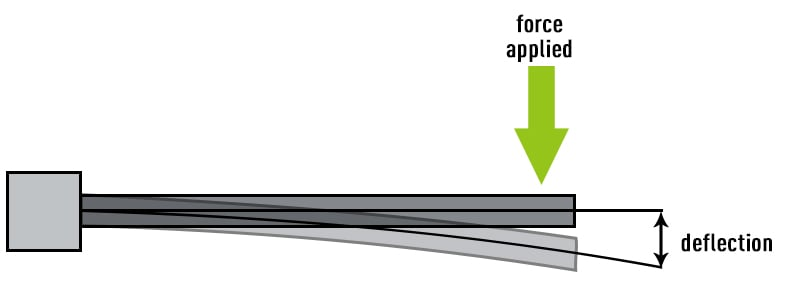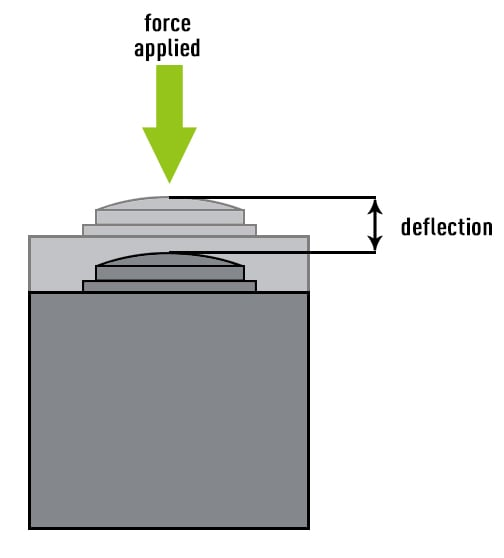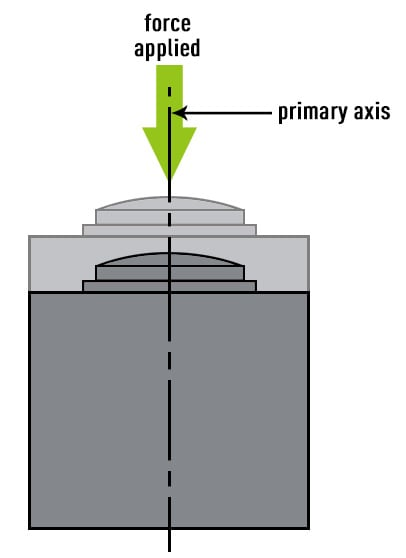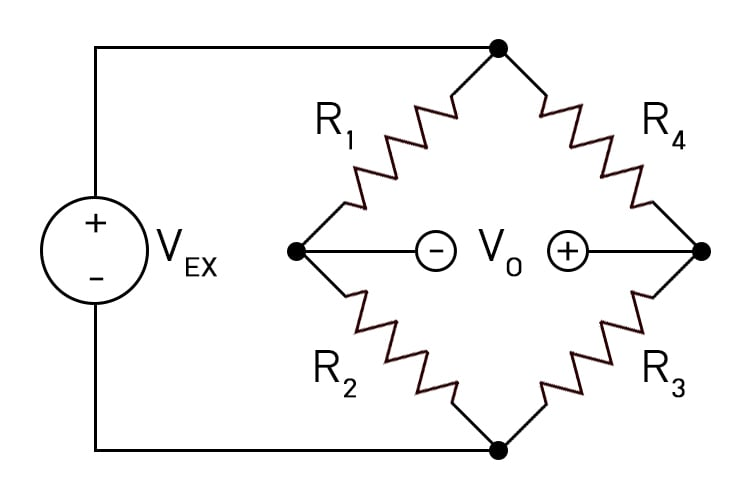Accuracy: Stated as a limit tolerance that defines the average deviation between actual output and theoretical output. In practical load cell applications, the potential errors of nonlinearity, hysteresis, non-repeatability and temperature effects do not normally occur simultaneously, nor are they necessarily cumulative. Therefore, accuracy is calculated based upon the RMS (root mean square) value of potential errors, assuming a defined temperature range, full rated load applied, and proper setup and calibration. Potential errors in other components of the measuring system are not included.

Calibration: The comparison of a load cell output signal against a known standard.

Calibration Curve: The comparison of load cell outputs to a known standard when output is measured between terminal points of zero (no load) and rated capacity.

Combined Error (Non-linearity and Hysteresis): The maximum deviation from the straight line drawn between the terminal points of zero (no-load) and rated capacity, usually expressed as a percentage of the rated output and measured on both increasing and decreasing loads.

Compensated Temperature Range: The range of temperature over which a load cell is compensated to maintain rated output and zero balance within specified limits.

Creep: The change in load cell output occurring with time (normally 30 minutes), while under load, and with all environmental conditions and other variables remaining constant. Usually measured with rated load applied and expressed as a percent of rated output over a specific period of time.

Dead (Static) Load: Also known as permanent or static loads. These are loads that are static over time, such as a frame, structure, or other immovable object.

Deflection: The change in length of the load cell along the primary axis between no-load and rated load conditions, with all other mechanical influences remaining constant; usually expressed in inches or millimeters.Drift: A random change in output under constant load conditions.

Dynamic Load: Non-static loading. It may involve considerations such as impact, momentum, vibration, slosh dynamics of fluids and similar phenomenon.

Error: The algebraic difference between the indicated and true value of the load being measured.

Excitation: The voltage applied to the input terminals of the load cell.

FM Approved:FM approval is the independent testing arm of international insurance carrier, FM Global. FM approvals use scientific research and testing to make sure products conform to the highest standards for safety and property loss prevention.

Hysteresis: The maximum difference between load cell output readings for the same applied load; one reading obtained by increasing the load from zero and the other by decreasing the load from rated output; usually measured at half rated output and expressed in percent of rated output.

Input Resistance: The resistance of the load cell bridge circuit measured at the positive and negative excitation terminals with no load applied and with the output terminals open-circuited.

Insulation (Leakage) Resistance: The DC resistance measured between three points: the transducers bridge circuit and the transducer element; the transducer bridge circuit and the cable shield; the transducer element and the cable shield. Note: Normally insulation resistance is measured with a minimum of fifty volts DC potential applied and under standard test conditions.

Load Cell: A precision, electromechanical spring element commonly constructed of alloy tool steel, stainless steel or an aluminum alloy that produces a low level voltage output signal proportional to an applied load, weight or force. For more information read our section on Strain Gauge Load Cell Basics.

mV/V: The full voltage output of the load cell expressed in terms of its supply voltage. E.g. 2mV/V load cell would produce 2mV times the supplied voltage at full capacity.

Non-linearity: The maximum deviation of the calibration curve from a straight line drawn between the no-load and rated outputs; usually expressed as a percentage of the rated output and measured on increasing load only.

NTEP:NTEP stands for National Type Evaluation Program, which is a non-profit corporation. Scales and other weighing equipment that are NTEP approved have been tested and evaluated to ensure they meet all government standards and requirements.

Operating Temperature Range: The extremes of temperature within which a load cell will operate without permanent adverse change to any of its performance characteristics.

Output: The electronic signal (voltage, current, etc.) produced by a load cell and measured as the algebraic difference between the signal at applied load and the signal at minimum load.

Output Resistance: The resistance of the transducer bridge circuit measured at the positive and negative signal terminals with no load applied and with the input terminals open-circuited.

PLC (Programmable Logic Controller): An industrial digital computer that has been adapted for the control of manufacturing processes.

Primary Axis: The axis along which the load cell is designed to be loaded; normally its geometric centerline.Rated Capacity: The maximum axial load that the load cell is designed to measure within its specifications.

Rated Output (Sensitivity, Full Scale Output): The algebraic difference of the load cell output at no-load compared to its output with the rated load applied; usually expressed in terms of millivolts/volt (mV/V) of excitation.

Recommended Excitation: The maximum excitation voltage of the transducer which operates within its specifications; usually expressed in VDC.

Reproducibility: The degree of agreement between the results of measurements taken under changing conditions.  The changing conditions may be due to different methods or instruments being used, measurements being made by different technicians, or measurements being made over a period of time.

Resistance (Electrical): A measure of the degree to which an object opposes the passage of an electric current. The standard primary unit of electrical resistance is the Ohm (Ω).

Safe Overload: The maximum load which can be applied without causing a permanent change in the performance specifications; usually expressed in percent of full scale capacity.

Shear Strain: is measured as a change in angle between lines that were originally perpendicular.

Shunt Calibration: Electrical simulation of load cell output by insertion of known shunt resistors between appropriate points within the circuitry.

Specification: The error limits within which a device should function for a given technical parameter. Also, an explicit set of requirements to be satisfied by a material, a design, and/or a product.

Strain Gauge: A device used to measure strain on an object. The most common type of strain gauge consists of an insulating flexible backing which supports a metallic foil pattern. The gauge is attached to the object by a suitable adhesive. As the object is deformed, the foil is deformed, causing its electrical resistance to change. This resistance change, usually measured using a Wheatstone bridge, is related to the strain by the quantity known as the gauge factor.Temperature Shift (Effect) on Output: The change in output due to a change in load cell temperature; usually expressed as a percentage of load per degree Fahrenheit (Celsius) change in temperature.

Temperature Shift (Effect) on Zero: The change in zero balance due to a change in ambient temperature; usually expressed in percent of rated output per degree Fahrenheit (Celsius) change in temperature.

Ultimate Overload: The maximum load which can be applied without causing structural failure; usually expressed in percent of full scale capacity.

Wheatstone Bridge: An electrical circuit used to measure an unknown electrical resistance by balancing two legs of a bridge circuit, one leg of which includes the unknown component. The Wheatstone Bridge circuit is nothing more than two simple series-parallel arrangements of resistances connected between a voltage supply terminal and ground producing zero voltage difference between the two parallel branches when balanced. A Wheatstone bridge circuit has two input terminals and two output terminals consisting of four resistors configured in a diamond-like arrangement.Zero Balance (Zero Offset): The output signal of the load cell with rated excitation and with no-load applied; the amount of deviation in output between true zero and a real load cell with zero load; usually expressed in percent of rated output.

Zero Return: The difference in zero balance measured immediately before rated load application of specified duration and measured after removal of the load, and when the output has stabilized.

Zero Shift: A permanent change in the no load output.

Zero Stability: The degree to which the transducer maintains its zero balance with all environmental conditions and other variables remaining constant.

Product Bulletins